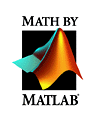MATLAB Web Server Demos

• MATLAB Matrix display creates a magic square (a square matrix in which all row, column, and diagonal sums are equal) using the MATLAB magic function and displays it in an HTML table. The HTML table is automatically generated by the MATLAB Web Server.
• Peaks Plot creates and displays a 3-D JPEG graphic created by the MATLAB peaks and surf functions. (peaks is a function of two variables, obtained by translating and scaling Gaussian distributions. surf produces a 3-D colored surface by plotting the colored parametric surface defined in this case by the results of the peaks function.)
• Softball Statistics reads statistics from a database and displays them in an automatically generated HTML table. This demo shows how the MATLAB Web Server can be invoked directly from a URL and how the results of a database (SQL, file, program, etc.) query can be retrieved using the MATLAB programming language.